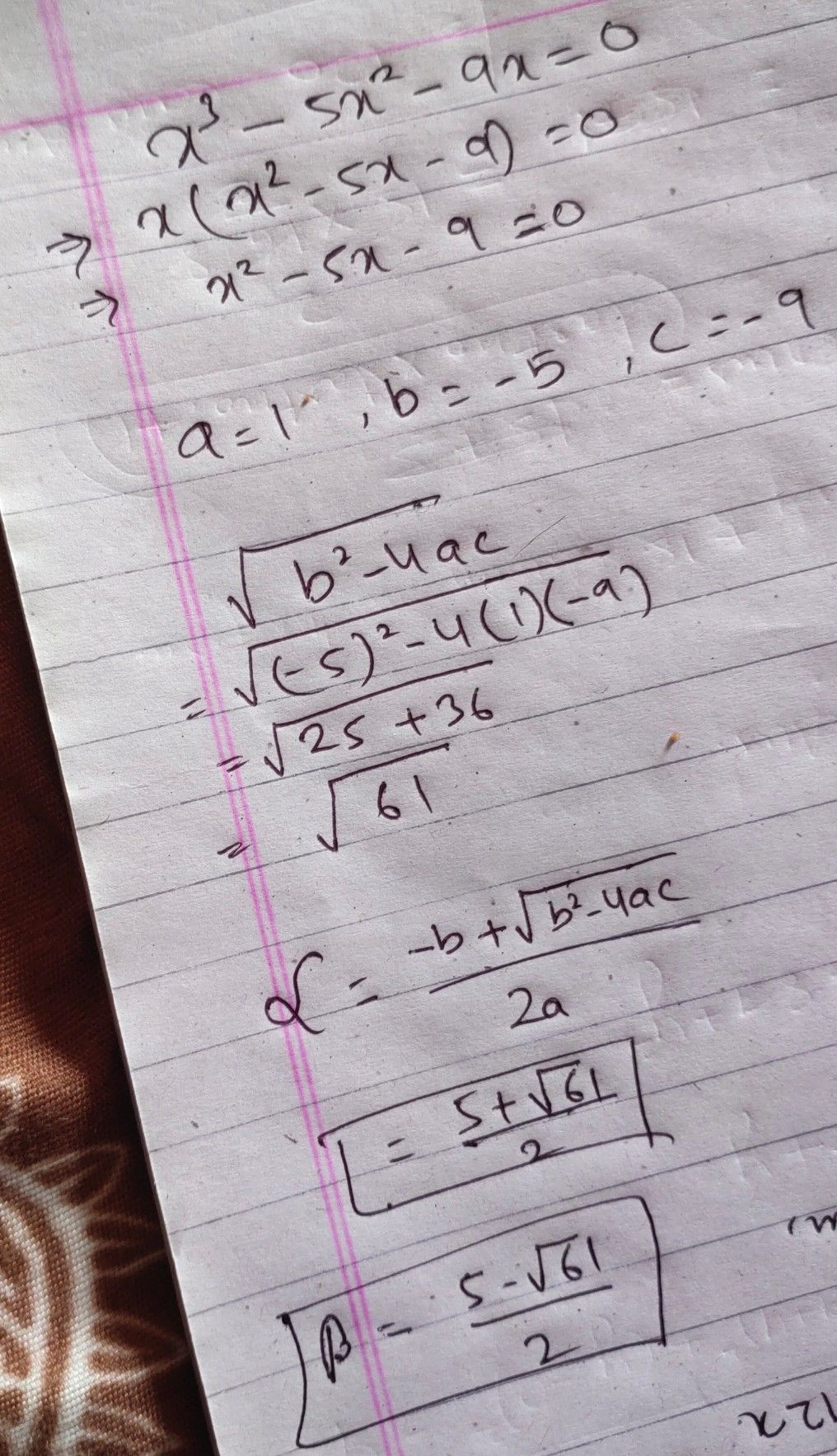Symbol
ProblemFor equations in quadratic $1c01100$ a he the quadratic formula to solve for the ro al This equation is normally used wh option. $111$ $3$ $x^{3}-5x^{2}-9x=0$ Get the ca of
Other
Question content